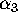Next: Integrated and single pulse Up: A Tutorial on Radio Previous: Conservation LawsLorentz Invariance

# Assignment 5

1. Find out the orbital parameters of the planets around PSR B1257+12
2. Why doesn't PSR B0655+64 follow the core mass radius relation
3. Estimate the change in the dispersion measure between orbital phases 0.30 and 0.40 for PSR B1957+20 (see the delay curve in the notes). Use your answer to estimate the change in the column density of electrons.
4. Estimate the orbital velocity of 2 neutron stars in a 2 hour orbit with a radius of 0.5 light seconds.
5. Evaluate the difference between the semi-major and semi-minor axis for a pulsar orbit that has an ececntricity of .
6. Describe what would happen, if you were riding a bicycle andwas large.

Jon Bell
Thu Dec 19 15:15:11 GMT 1996